# Local ring

Jump to: navigation, search

A commutative ring with a unit that has a unique maximal ideal. Ifis a local ring with maximal ideal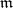, then the quotient ringis a field, called the residue field of.

Examples of local rings. Any field or valuation ring is local. The ring of formal power series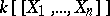over a fieldor over any local ring is local. On the other hand, the polynomial ringwithis not local. Letbe a topological space (or a differentiable manifold, an analytic space or an algebraic variety) and letbe a point of. Letbe the ring of germs atof continuous functions (respectively, differentiable, analytic or regular functions); thenis a local ring whose maximal ideal consists of the germs of functions that vanish at.

Some general ring-theoretical constructions lead to local rings, the most important of which is localization (cf. Localization in a commutative algebra). Letbe a commutative ring and let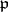be a prime ideal of. The ring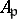, which consists of fractions of the form, where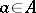,, is local and is called the localization of the ringat. The maximal ideal ofis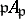, and the residue field ofis identified with the field of fractions of the integral quotient ring. Other constructions that lead to local rings are Henselization (cf. Hensel ring) or completion of a ring with respect to a maximal ideal. Any quotient ring of a local ring is also local.

A property of a ring(or an-module, or an-algebra) is called a local property if its validity for(or, or) is equivalent to its validity for the rings(respectively, modules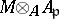or algebras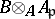) for all prime idealsof(see Local property).

The powers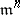of the maximal idealof a local ringdetermine a basis of neighbourhoods of zero of the so-called local-ring topology (or-adic topology). For a Noetherian local ring this topology is separated (Krull's theorem), and any ideal of it is closed.

From now on only Noetherian local rings are considered (cf. also Noetherian ring). A local ring is called a complete local ring if it is complete with respect to the-adic topology; in this case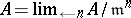. In a complete local ring the-adic topology is weaker than any other separated topology (Chevalley's theorem). Any complete local ring can be represented as the quotient ring of the ring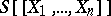of formal power series, whereis a field (in the case of equal characteristic) or of a complete discrete valuation ring (in the case of different characteristic). This theorem makes it possible to prove that complete local rings have a number of specific properties that are absent in arbitrary Noetherian local rings (see ); for example, a complete local ring is an excellent ring.

A finer quantitative investigation of a local ringis connected with the application of the concept of the adjoint graded ring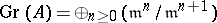. Let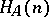be the dimension of the vector space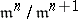over the residue field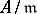; as a function of the integer argumentit is called the Hilbert–Samuel function (or characteristic function) of the local ring. For largethis function coincides with a certain polynomial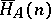in, which is called the Hilbert–Samuel polynomial of the local ring(see also Hilbert polynomial). This fact can be expressed in terms of a Poincaré series: The formal series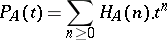is a rational function of the form, whereis a polynomial and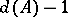is the degree of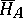. The integer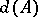is the (Krull) dimension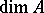of the ringand is one of the most important invariants of a ring. Moreover,is equal to the least number of elementsfor which the quotient ring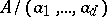is Artinian (cf. Artinian ring). If these elements can be chosen in such a way that they generate the maximal ideal, thenis called a regular local ring. The regularity ofis equivalent to the fact that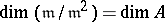. For a-dimensional regular ring,and. Geometrically, regularity means that the corresponding point of the (analytic or algebraic) variety is non-singular.

Besides the characteristic function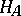and the dimension and multiplicity connected with it, a local ring has various invariants of a homological kind. The main one of these is the depth(see Depth of a module); the condition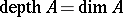distinguishes among local rings the so-called Cohen–Macaulay rings (cf. Cohen–Macaulay ring). It is not known (1989) whether there is a modulewith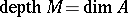for an arbitrary or a complete local ring. Other homological invariants are the so-called Betti numbers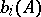of a local ring, that is, the dimensions of the-spaces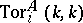, whereis the residue field of. The question of the rationality of the Poincaré series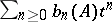is open, although for many classes of rings an affirmative answer is known. There are also invariants of an algebraic-geometrical nature; for their definition one uses resolution of the singularity corresponding to the local ring.

A similar theory has been constructed for semi-local rings; that is, rings that have finitely many maximal ideals. The role of a maximal ideal for them is played by the Jacobson radical.